##### Calculus Workbook For Dummies with Online Practice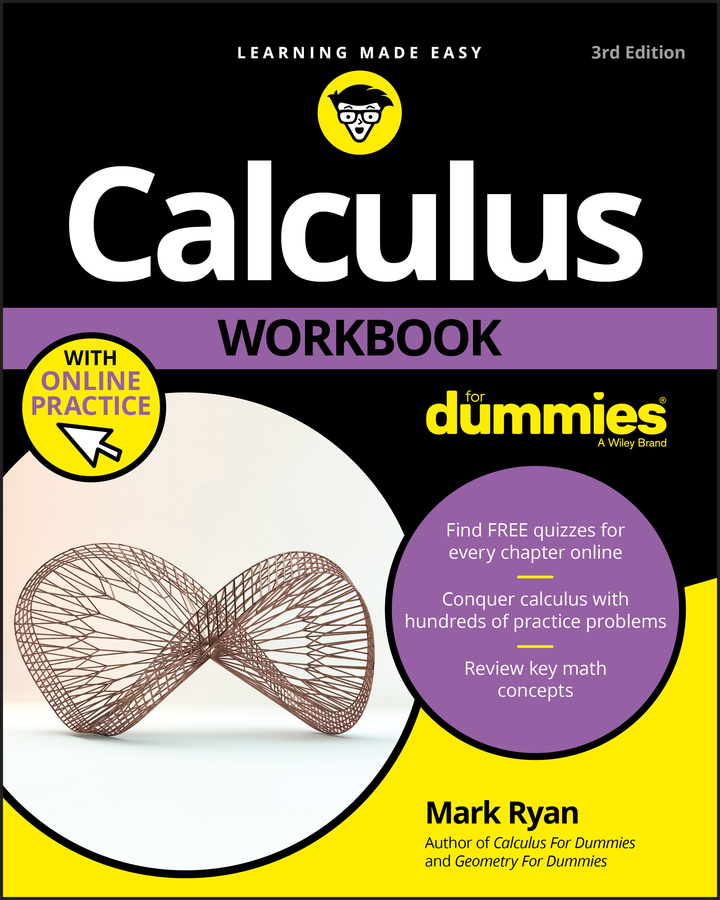L’Hôpital’s rule is a great shortcut for doing some limit problems. (And you may need it someday to solve some improper integral problems, and also for some infinite series problems.)

As with most limit problems — not counting no-brainer problems — you can’t do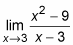with direct substitution: plugging 3 into x gives you 0/0, which is undefined. The algebraic way of doing the limit is to factor the numerator into (x – 3)(x + 3) and then cancel the (x – 3). That leaves you with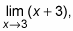which equals 6.

Now watch how easy it is to take the limit with L’Hôpital’s rule. Simply take the derivative of the numerator and denominator. Don’t use the quotient rule; just take the derivatives of the numerator and denominator separately. The derivative of x2 – 9 is 2x and the derivative of x – 3 is 1. L’Hôpital’s rule lets you replace the numerator and denominator by their respective derivatives like this: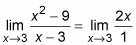The new limit is a no-brainer: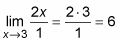That’s all there is to it. L’Hôpital’s rule transforms a limit you can’t do with direct substitution into one you can do with substitution. That’s what makes it such a great shortcut.

Here’s the mathematical mumbo jumbo.

L’Hôpital’s rule: Let f and g be differentiable functions.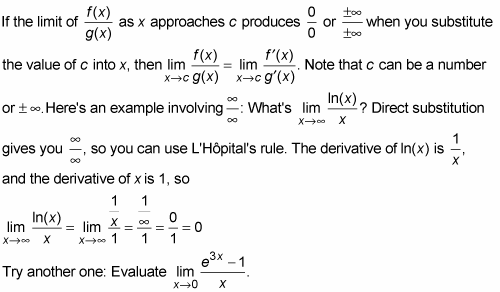Substitution gives you 0/0 so L’Hôpital’s rule applies.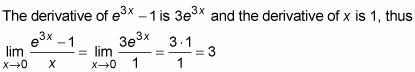Keep in mind that to use L’Hôpital’s rule, substitution must produce either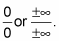You must get one of these acceptable “indeterminate” forms in order to apply the shortcut. Don’t forget to check this.